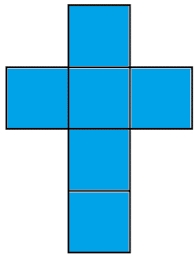# Edges or sides

Calculate the cube volume, if the sum of the lengths of all sides is 276 cm.

V =  12167 cm3

### Step-by-step explanation:Did you find an error or inaccuracy? Feel free to write us. Thank you!Tips to related online calculators
Tip: Our volume units converter will help you with the conversion of volume units.

## Related math problems and questions:

• Cube 7Calculate the volume of a cube, whose sum of the lengths of all edges is 276 cm.
• Sum of the edgesThe sum of the lengths of all edges of the cube is 72 cm. How many cm2 of colored paper are we going to use for sticking?
• Cube edgesThe sum of the lengths of the cube edges is 42 cm. Calculate the surface of the cube.
• The cubeThe cube has a surface of 600 cm2. What is its volume?
• The cubeThe cube has a surface area of 486 m ^ 2. Calculate its volume.
• CubeThe sum of lengths of cube edges is 57 cm. What is its surface and volume?
• Three cubesTwo cube-shaped boxes with edges a = 70 cm; b = 90 cm must be replaced by one cube-shaped box. What will be its edge?
• Cuboid edges in ratioCuboid edges lengths are in ratio 2:4:6. Calculate their lengths if you know that the cuboid volume is 24576 cm3.
• Cube V2SThe volume of the cube is 27 dm cubic. Calculate the surface of the cube.
• CubeThe sum of all cube edges is 30cm. Find the surface area of the cube.
• Cube surfce2volumeCalculate the volume of the cube if its surface is 150 cm2.
• Cuboid surfaceDetermine surface area of cuboid if its volume is 52.8 cm cubic and length of the two edges are 2 cm and 6 cm.
• Cube basicsHow long is the edge length of a cube with volume 15 m3?
• Cube 1-2-3Calculate the volume and surface area of the cube ABCDEFGH if: a) /AB/ = 4 cm b) perimeter of wall ABCD is 22 cm c) the sum of the lengths of all edges of the cube is 30 cm.
• Surface of cuboidFind the surface of the cuboid if its volume is 52.8 cm3 and the length of its two edges is 2 cm and 6 cm.
• Cube edgeDetermine the edges of the cube when the surface is equal to 37.5 cm square.
• Cuboid to cubeA cuboid with dimensions of 9 cm, 6 cm, and 4 cm has the same volume as a cube. Calculate the surface of this cube.